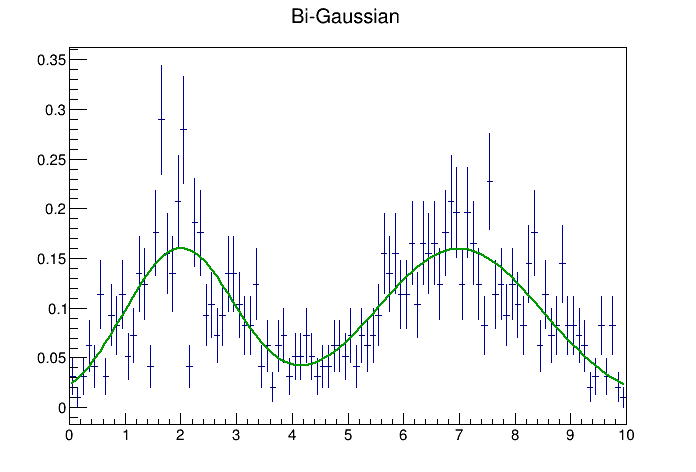# exampleTKDE¶

Example of using the TKDE class (kernel density estimator)

Author: Lorenzo Moneta, Bartolomeu Rabacal (Dec 2010)
This notebook tutorial was automatically generated with ROOTBOOK-izer from the macro found in the ROOT repository on Wednesday, August 17, 2022 at 09:34 AM.

In :
%%cpp -d
#include "TH1.h"
#include "TF1.h"
#include "TKDE.h"
#include "TCanvas.h"
/*#include "TStopwatch.h"*/
#include "TRandom.h"
#include "Math/DistFunc.h"
#include "TLegend.h"


Arguments are defined.

In :
int n = 1000;


generate some gaussian points

In :
int nbin = 100;
double xmin = 0;
double xmax = 10;

TH1D * h1 = new TH1D("h1","h1",nbin,xmin,xmax);


generate some points with bi- gaussian distribution

In :
std::vector<double> data(n);
for (int i = 0; i < n; ++i) {
if (i < 0.4*n) {
data[i] = gRandom->Gaus(2,1);
h1->Fill(data[i]);
}
else {
data[i] = gRandom->Gaus(7,1.5);
h1->Fill(data[i]);
}
}

input_line_54:2:2: warning: 'data' shadows a declaration with the same name in the 'std' namespace; use '::data' to reference this declaration
std::vector<double> data(n);
^


scale histogram

In :
h1->Scale(1./h1->Integral(),"width" );
h1->SetStats(false);
h1->SetTitle("Bi-Gaussian");
h1->Draw();

Info in <TCanvas::MakeDefCanvas>:  created default TCanvas with name c1


drawn true normalized density

In :
TF1 * f1 = new TF1("f1","0.4*ROOT::Math::normal_pdf(x,1,2)+0.6*ROOT::Math::normal_pdf(x,1.5,7)",xmin,xmax);
f1->SetLineColor(kGreen+2);
f1->Draw("SAME");


create TKDE class

In :
double rho = 1.0; //default value
TKDE * kde = new TKDE(n, &data, xmin,xmax, "", rho);

input_line_62:3:27: error: reference to 'data' is ambiguous
TKDE * kde = new TKDE(n, &data, xmin,xmax, "", rho);
^
input_line_54:2:22: note: candidate found by name lookup is '__cling_N523::data'
std::vector<double> data(n);
^
/usr/include/c++/9/bits/range_access.h:318:5: note: candidate found by name lookup is 'std::data'
data(initializer_list<_Tp> __il) noexcept
^
/usr/include/c++/9/bits/range_access.h:289:5: note: candidate found by name lookup is 'std::data'
data(_Container& __cont) noexcept(noexcept(__cont.data()))
^
/usr/include/c++/9/bits/range_access.h:299:5: note: candidate found by name lookup is 'std::data'
data(const _Container& __cont) noexcept(noexcept(__cont.data()))
^
/usr/include/c++/9/bits/range_access.h:309:5: note: candidate found by name lookup is 'std::data'
data(_Tp (&__array)[_Nm]) noexcept
^


kde->Draw("[email protected] Same");

In :
kde->Draw("SAME");

TLegend * legend = new TLegend(0.6,0.7,0.9,0.95);
legend->Draw();

input_line_64:2:3: error: use of undeclared identifier 'kde'
(kde->Draw("SAME"))
^
Error in <HandleInterpreterException>: Error evaluating expression (kde->Draw("SAME"))
Execution of your code was aborted.


Draw all canvases

In :
gROOT->GetListOfCanvases()->Draw()